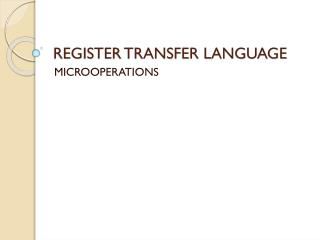DownloadDownload PresentationREGISTER TRANSFER LANGUAGE

# REGISTER TRANSFER LANGUAGE

Download Presentation## REGISTER TRANSFER LANGUAGE

- - - - - - - - - - - - - - - - - - - - - - - - - - - E N D - - - - - - - - - - - - - - - - - - - - - - - - - - -
##### Presentation Transcript

1. REGISTER TRANSFER LANGUAGE MICROOPERATIONS

2. TODAY OUTLINES • Logic Microoperations • Shift Microoperations

3. LOGIC MICROOPERATIONS

4. Logic Microoperations LOGIC MICROOPERATIONS • Specify binary operations on the strings of bits in registers • Logic microoperations are bit-wise operations, i.e., they work on the individual bits of data • useful for bit manipulations on binary data • useful for making logical decisions based on the bit value • The symbols for the four basic logic operations are: • OR (), • AND (), • XOR (), • Complement/NOT

5. LOGIC MICROOPERATIONS Example: R3 R4 v R6 • v OR (SETS Bits) • ^ AND (CLEARS Bits) •  XOR (Complement Bits, 2 Sources) • NOT (Complement Bits, 1 Source) Example: R2 DR ^ R1 Example: PC PC  DR Example: R6 R1

6. LOGIC MICROOPERATIONS Example: • Let R1 = 10101010 and R2 = 11110000 • After the operations, R0 becomes:

7. Logic Microoperations HARDWARE IMPLEMENTATION OF LOGIC MICROOPERATIONS A i 0 B i 1 4 X 1 F i MUX 2 3 Select S 1 S 0 Function table -operation S1 S0 Output 0 0 F = A  B AND 0 1 F = AB OR 1 0 F = A  B XOR 1 1 F = A’ Complement

8. APPLICATIONS OF LOGIC MICROOPERATIONS • Logic microoperations can be used to manipulate individual bits or a portions of a word in a register • Logic microoperations can change bit values, clear a group of bits, or insert new bit values into a register.

9. AND microoperation • Used for clearing one or more bits in a register to 0. • Boolean equations: • X • 0 = 0 When ANDed with 0, binary variable X produces 0. • X • 1 = X When ANDed with 1, binary variable X remains unchanged.

10. AND microoperation X • 0 = 0 X • 1 = X Example: 10101101 10101011 R1 (data) 00000000 11111111 R2 (mask) 00000000 10101011 R1  R1 ^ R2 AND operation: • Selectively clear bits of a register • Masking out the bits R1 : High-order byte = Clear Low-order byte = Unchanged

11. OR microoperation • Used to set one or more bits in a register. • Boolean equations: • X + 1 = 1 When ORed with 1, binary variable X produces a 1. • X + 0 = X When ORed with 0, binary variable X remains unchanged.

12. OR microoperation • X + 1 = 1 • X + 0 = X Example: 10101101 10101011 R1 (data) 11111111 00000000 R2 (mask) 11111111 10101011 R1  R1 v R2 OR operation: • Selectively set bits of a register R1: High-order byte = Set to 1’s Low-order byte = Unchanged

13. XOR microoperation • Used to complement one or more bits in a register. • Boolean equations: • X 1 = X When XORed with 1, binary variable X complemented. • X 0 = X When XORed with 0, binary variable X remains unchanged.

14. XOR microoperation • X 1 = X • X 0 = X Example: 10101101 10101011 R1 (data) 11111111 00000000 R2 (mask) 01010010 10101011 R1  R1R2 XOR operation: • Selectively complement bits of a register R1: High-order byte = Complemented Low-order byte = Unchanged

15. SHIFT MICROOPERATIONS

16. LSB 7 6 5 4 3 2 1 0 SHIFT MICROOPERATIONS • Shift microoperations are used for: • Lateral movement of data. • Serial transfer of data. • Manipulating the contents of registers in arithmetic, logical, and control operations. • Left shift : toward the MSB • Right shift : toward the LSB MSB

17. Shift Microoperations SHIFT MICROOPERATIONS • There are three types of shifts • Logical shift • Circular shift • Arithmetic shift • What differentiates them is the information that goes into the serial input/incoming bit • A right shift operation • A left shift operation LSB Serial input Serial input MSB

18. Shift Microoperations SHIFT MICROOPERATIONS • A right shift operation • A left shift operation LSB Serial input MSB Serial input

19. SHIFT MICROOPERATIONS • In RTL, string of letters are used to specify 1-bit shiftoperations: • Shift left : sl • Shift right : sr

20. Shift Microoperations 0 0 LOGICAL SHIFT • Assume the serial input/incoming bit to the shift is a 0. • A right shift operation: • A left shift operation: • Example of Shifts: Type Symbolic Source R2 After Shift: Designation Destination R1 Shift Left R1 sl R2 10011110 00111100 Shift Right R1 sr R2 11100101 01110010

21. SHIFT MICROOPERATIONS Example 1: • Let R2 = 11001001 • After the Shift operation, R1 becomes: Note: These shifts "zero fill". Sometimes a separate flip-flop is used to provide the data shifted in, or to “catch” the data shifted out.